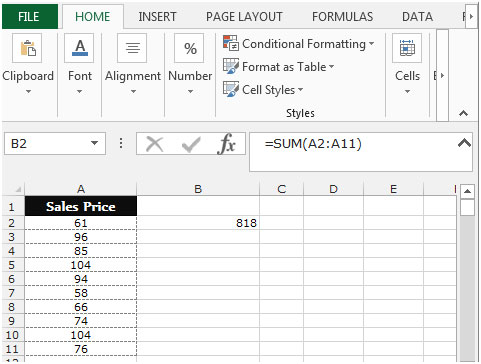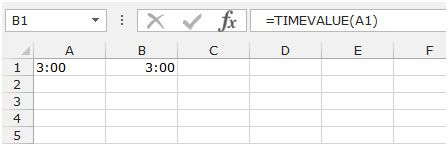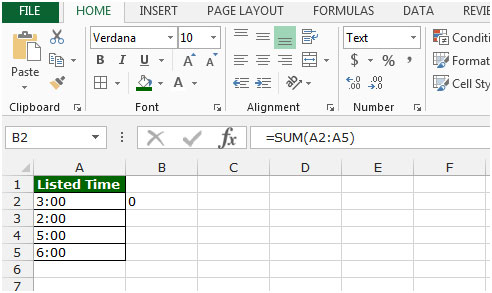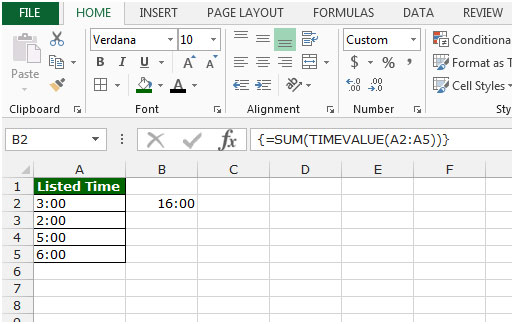# Adding Text Values Representing Time in Microsoft Excel 2010

To add text values representing time, we use the Sum function along with Time value function in Microsoft Excel 2010.

SUM:- Sum function is used for adding all the numbers in a range or cells.

The syntax of SUM formula:-  =SUM(number1, number2…..)

For example: We have data in range A2:A11 and we want to return the total amount.

To return the total amount, follow below given steps:-

• Write the formula in cell B2.
• =SUM(A2:A11)
• Press Enter on your keyboard.
• The function will return the total value.TIMEVALUE:- To convert a text time to an excel serial number for a time, a number from 0 (12:00:00 Am) to 0.999988426 (11:59:59 PM). Format the number with a time format after entering the formula.

The syntax of TIMEVALUE formula:-  =TIMEVALUE(time_text)

For example:- Cell A1 contains the time as text, and we want to convert it into time value.

• Write the formula in cell B1
• =TIMEVALUE(A1)
• Press Enter on your keyboard.
• The function will convert the text in to time format.Let’s take an example to understand how we can add text values representing time.

The times listed in column A are formatted as text when trying to add them using a simple formula =SUM(A2:A6), a false result 0 is returned.How can we add text values correctly?

• Write the formula in cell B2.
• =SUM(TIMEVALUE(A2:A5))
• Press Ctrl+Shift+Enter on your keyboard to create an array formula.
• {=SUM(TIMEVALUE(A2:A5))}
• The function will add the text values representing the times.This is the way we can add the text values representing time in Microsoft Excel.

Terms and Conditions of use

The applications/code on this site are distributed as is and without warranties or liability. In no event shall the owner of the copyrights, or the authors of the applications/code be liable for any loss of profit, any problems or any damage resulting from the use or evaluation of the applications/code.